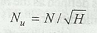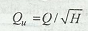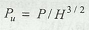# Unit Speed, Unit Discharge and Unit Power

What is Unit Speed, Unit Discharge and Unit Power of a turbine? How do we calculate these using formula?

The unit speed is the speed of the turbine operating under one meter head. Mathematically, unit speed,The unit discharge is the discharge through a turbine when the head on the turbine is unity. Mathematically, unit discharge,The unit power is the power developed by a turbine when the head on the turbine is unity. Mathematically, unit power,### You may like these posts:

1.2.how it is derived?
what is the proof?
if it is per head power/discharge/speed then why root signs?

1.These are empirical relations and have no proofs.

3.Hou to find dedication for above this formula ?👍,
Unit speed,unit discharge,unit power any person can u explain. Briefly ?

4.The unit speed (Nu) of a turbine is given by the expression ????